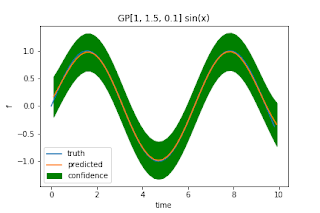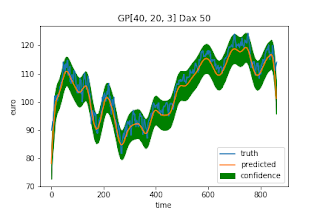## Saturday, July 28, 2018

### Simple Gaussian Processes For Regression

In this post I am implementing a simple Gaussian Process Regressor. A Gaussian Process is a
probabilistic kernel model. Basically I am following the "Machine Learning: A probabilistic Perspective" book by Kevin Murphy.

### The Basics

For an input $x_i$ and output $y_i = f(x_i)$, infer a distribution over functions of the data:
$p(f | X, y)$. In order to make predictions $y*$ for a new data point $x*$ given all the previous observations, we define:

$p(y*|x*, X, y) = \int p(y*|f, x*) p(f|x, y) df$

For our data $X = x_1 ... x_n$ the Gaussian process assumption is that the the distributions over functions $p(f_1 ... f_N)$ is jointly Gaussian with mean $\mu(x)$ and a covariance function $\Sigma_{ij} = k(x_i, x_j)$ and a kernel $k$.

### Covariance structure

It is quite common to leaf the mean function at zero. Given a kernel, we need to compute the covariance function between all training and test instances. However, we structure the kernel matrix in the following way.

The sub-matrix $K$ describes the affinity between all training instances and the sub-matrix $K*$ describes the affinity between training and test instances. Finally, the submatrix $K**$ describes the affinity between test instances to itself. The image above shows the final covariance structure. With that, our function $f*$ can be inferred as: $(f, f*) ~ N(0, \Sigma)$.

In order to obtain the prediction calculate: $f* = K_* \bullet K^{-1} \bullet f$.
If we want the find the variance of the predictions we calculate: $trace(K_{**} - K_* \bullet K^{-1} \bullet K_*^T)$

In my opinion the method is very similar to support vector machines and to instance beased learning methods in general. The difference is that a SVM is only using a small set of training examples called support vectors, while the Gaussian process compares every example to all others in order to make a prediction.

### A Naive Gaussian Process

Below I implemented a naive Gaussian process using numpy. Basically, I am building the three components of the covariance structure and then solve the prediction equations above. I call my method naive since computing the inverse has a worst case complexity of $O(n^3)$
So it is not advisable to use this method for larger datasets.
class GaussianProcess:

def __init__(self, kernel):
self.kernel = kernel

def predict(self, train_x, train_y, test_x):
(n, _) = train_x.shape
(m, _) = test_x.shape
covar_train      = np.zeros((n, n))
covar_train_test = np.zeros((n, m))
covar_test       = np.zeros((m, m))
for i in range(0, n):
for j in range(0, n):
covar_train[i, j] = self.kernel.k(train_x[i], train_x[j], i == j)
for i in range(0, m):
for j in range(0, m):
covar_test[i, j] = self.kernel.k(test_x[i], test_x[j], i == j)
for i in range(0, n):
for j in range(0, m):
covar_train_test[i, j] = self.kernel.k(train_x[i], test_x[j], False)
covar_inv  = np.linalg.inv(covar_train)
prediction = np.dot(np.dot(covar_train_test.T, covar_inv), train_y)
confidence = covar_test - np.dot(covar_train_test.T, np.dot(covar_inv, covar_train_test))
confidence = np.sqrt(np.array([confidence[i, i] for i in range(0, m)]))
return prediction, confidence


### Squared Exponential Kernel

For my experiments I use a squared exponential kernel and also add a variance parameter for for noisy observations:

$k(x_i, x_j) = \sigma_f^2 e^{-\frac{1}{2l^2} || x_i - x_j ||_2^2} + \sigma_y^2 \mathbb{I}(i == j)$

The parameters are as follows:
• $\sigma_f^2$: Variance giving the scale of the output
• $\sigma_y^2 \mathbb{I}(i == j)$: Noise variance applied to diagnoal
• $l$: Scale on input
again, the implementation is quite simple in numpy.
class SquaredExponential:

def __init__(self, variance, alpha, noise):
self.alpha = alpha
self.variance = variance
self.noise = noise
self.scaler = -1.0 / (2 * alpha**2)

def k(self, a, b, on_diag):
error  = np.sum(np.square(a - b))
f      = np.exp(self.scaler * error)
scaled = self.variance * f
if on_diag:
scaled += self.noise
return scaled


### Results on some simple example datasets

I tried my method on three datasets, 2 times, I am intepolating stock marktet data and one time a sine wave. Each time, the index is the input to the method and the output for the stock market prediction is the closing price.
Sine Wave Interpolation:Stock Interpolation Dax:Stock Interpolation US:## Saturday, July 21, 2018

### Financial Machine Learning Experiement

As a fun project, I tried to predict the stock market and implemented a small library todo so.
Basically, I want to use a sliding window of historic prices of several companies or funds and
predict the price of a single company. In the end, I achieve decent results in a 10 day window and
predicting 5 days into the future. However, my error is still several euros large :)

The code can be found here: [:Github:]

### Learning Problem, Features and Modeling

First we define the return of interest (ROI) as:

$roi(t, t + 1) = \frac{x_t - x_{t + 1}}{x_t}$

Which is the percentage of earnings at a time $t + 1$ measured to a previous investment
at $t$. In order to extract the features, we compute sliding windows over our historic prices.
For each sample in a window, we compute the ROI to the start of the window, which represents
the earnings to the start of the window. For a window of $T$ steps and $n$ stocks, we flatten the sliding windows and get a feature vector of size $T x n$ of ROI entries. Our target variable we want
to predict is a stock price $d$ days after the last day of the window. The traget is converted
to the ROI, too. So we try to predict the earnings after $d$ days after the last day of the window
which represents a potential investment.Red: roi in the window, Blue: ROI for prediction

### Some Experimental Results

First we downloaded several historic price datasets from yahoo finance and load them using pandas. We take the date column as the index and interpolate missing values:

data = [
]


We learn the following classifier on our data.

predictors = [
('RF', RandomForestRegressor(n_estimators=250)),
('GP', GaussianProcessRegressor(kernel=RBF(length_scale=2.5))),
('NN', KNeighborsRegressor(n_neighbors=80)),
('NE', KerasPredictor(model, 10, 512, False)),
]


The neural network's architecture (named model above):

hidden = [256, 128, 64, 32]
inp    = (len(data.stoxx) * WIN,)
model  = Sequential()